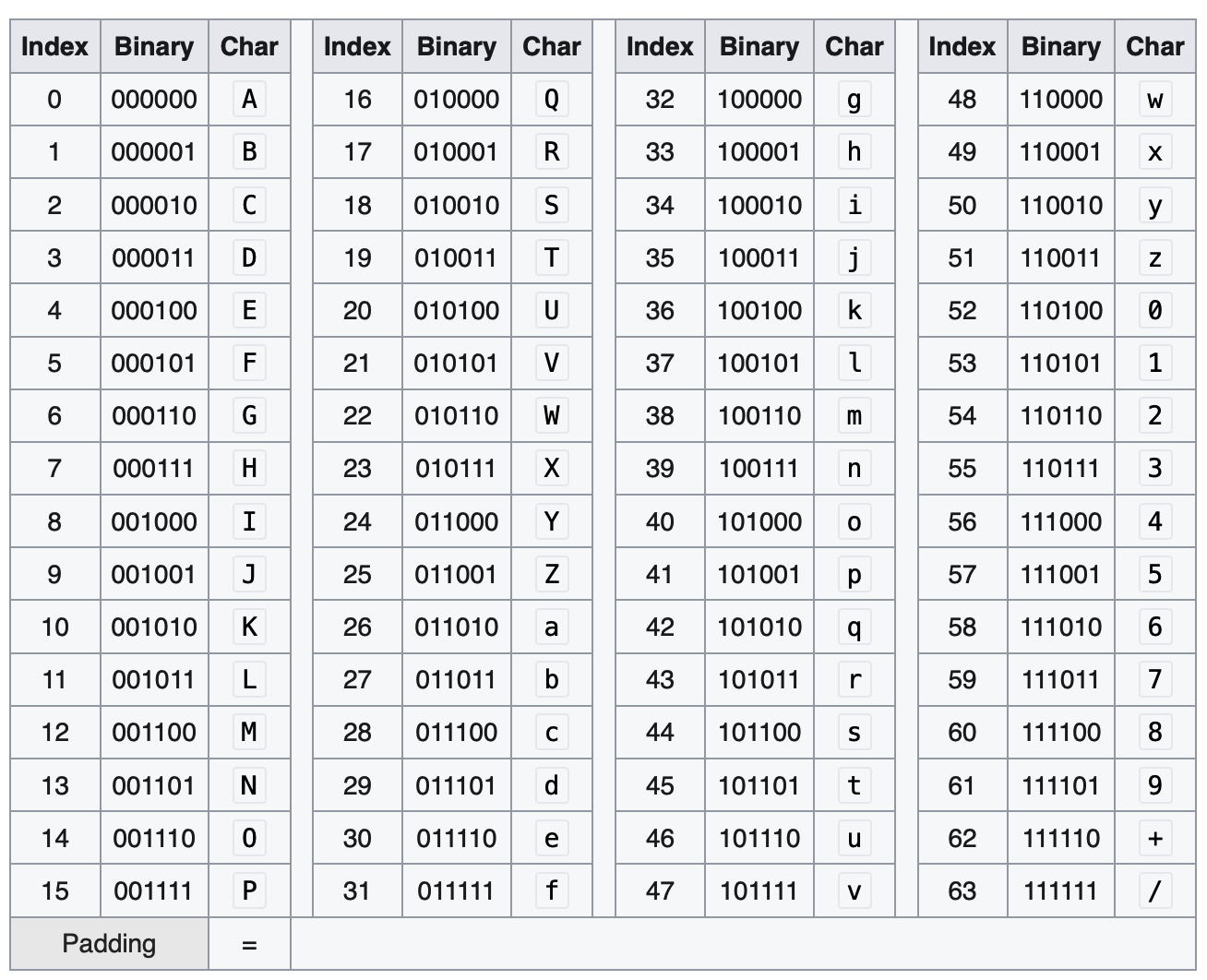# Base64 in python

Posted by chunyang on March 18, 2022

Base64 encode and decode are used in many places. What is base64 and how to implement base64 encode and decode in python?

## What is base64?

Base64

Wikipedia says it is a binary to text encodidng method. Base64, like a decimal system on base 10, is based on 64.

6 digits is used to represent a number from 0-63. So basically in base64, it uses 4 characters to represent 3 bytes. The memory increase will be more than 33% (allowing for padding).

Following is a picture of the base64 number and its corresponding characters.So in summary:

• It is a system based on 64
• 4 characters to represent 3 bytes, memory increase will be at least 33%

## How to encode bytes into base64 in python?

### Python standard library

import base64

content = b"Man"

result = base64.b64encode(content)
print(result.decode("utf-8"))

# "TWFu"


### Make your hands dirty

import base64

def base64_code_book():
code_book = {}
A = ord("A")
Z = ord("Z")
a = ord("a")
z = ord("z")
zero = ord("0")
nine = ord("9")

Cur = 0
N = Z - A + 1
code_book.update((n, chr(c)) for n, c in zip(range(Cur, Cur+N+1), range(A, Z+1)))

Cur += N
N = z - a + 1
code_book.update((n, chr(c)) for n, c in zip(range(Cur, Cur+N+1), range(a, z+1)))

Cur += N
N = nine - zero + 1
code_book.update(
(n, chr(c)) for n, c in zip(range(Cur, Cur+N+1), range(zero, nine+1))
)

code_book = "+"
code_book = "/"

def b64encode(content):
binary = ""
code_book, padding = base64_code_book()
for c in content:
b = f"{c:08b}"
binary += b

print(binary)
size = len(binary)
step = 24
result = ""
for start in range(0, size, step):
end = min(start+step, size)
diff = end - start
if diff < 24:
# Padding needed here
if diff < 10:
# one byte, padding 2
result += code_book[int(binary[start : start+6], base=2)]
result += code_book[int(binary[start+6:]+"0000", base=2)]
else:
# two byte, padding one
result += code_book[int(binary[start : start+6], base=2)]
result += code_book[int(binary[start+6 : start+12], base=2)]
result += code_book[int(binary[start+12:]+"00", base=2)]
else:
for _ in range(0, 24, 6):
result += code_book[int(binary[_+start : _+start+6], base=2)]
return result.encode("utf-8")

content = b"Man"
res1 = b64encode(content)
res2 = base64.b64encode(content)
print(f"{res1}")
print(f"{res2}")
print(f"{res1==res2}")

content = b"Many hands make light wor"
res1 = b64encode(content)
res2 = base64.b64encode(content)
print(f"{res1}")
print(f"{res2}")
print(f"{res1==res2}")

• I dont know why base64.b64encode returns type of bytes?

## How to decode a base64 encoded string?

### Python standard library

import base64

b = b"TWFu"
res = base64.b64decode(b)
print(res.decode("utf-8")


### Make your hands dirty again

def b64decode(content):
content = content.decode("utf-8")
size = len(content)
print(content)
code_book, padding = base64_code_book()
reverse_code_book = dict((v, k) for k, v in code_book.items())
step = 4
binary = ""
for start in range(0, size, step):
end = start + 4
shift = 0
while content[end-1] == padding:
shift += 1
end -= 1
temp = ""
for c in content[start:end]:
v = reverse_code_book[c]
temp += f"{v:06b}"
if shift != 0:
binary += temp[:-shift*2]
else:
binary +=  temp
step = 8
size = len(binary)
print(f"Binary: {binary}")
result = b""
for start in range(0, size, step):
result += int(binary[start:start+8], base=2).to_bytes(1, byteorder=sys.byteorder)
return result

content = b"Man"
print(b64decode(b64encode(content)))


Please refer to previous paragraph for the definition of b64encode

## Thoughts

### Convert from ascii and int

In cpp, the language allows us to directly do mathematical operations between char and int. In python, we can not.

chr and ord can be used to convert between char and int.

b = ord('a')
a = chr(b)


### Convert an int to binary representation

Python provides function like bin, oct and hex to convert from a int to its corresponding format. What if we want to control the length of the binary representation?

a = 3
b = bin(a) # 0b101
c = b[2:].rjust(8, '0') # c = b[2:].zfill(8) # only fill zero
d = b[2:].ljust(8, '0')

e = f"{a:0>6b}"
f = f"{a:0<6b}"
g = f"{a:0^6b}" # Fill left and  right


### Int to bytes

a = 3
length = 1
print(int.to_bytes(1, a))

### bytes array